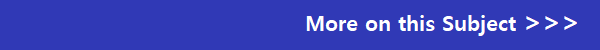## Eduqas GCSE Maths Past Papers

The Eduqas GCSE (9-1) Maths past exam papers section of Revision Maths. You can download the papers and marking schemes by clicking on the links below.

June 2022 Eduqas New GCSE (9-1) Mathematics Past Papers (C300U)

Mathematics - Component 1: Non Calculator Mathematics (Foundation Tier) (C300U10-1)

Mathematics - Component 1: Non Calculator Mathematics (Higher Tier) (C300UA0-1)

Mathematics - Component 2: Calculator- Allowed Mathematics (Foundation Tier) (C300U20-1)

Mathematics - Component 2: Calculator- Allowed Mathematics (Higher Tier) (C300UB0-1)

November 2020 Eduqas New GCSE (9-1) Mathematics Past Papers (C300U)

Mathematics - Component 1: Non Calculator Mathematics (Foundation Tier) (C300U10-1)

Mathematics - Component 1: Non Calculator Mathematics (Higher Tier) (C300UA0-1)

Mathematics - Component 2: Calculator- Allowed Mathematics (Foundation Tier) (C300U20-1)

Mathematics - Component 2: Calculator- Allowed Mathematics (Higher Tier) (C300UB0-1)

November 2019 Eduqas New GCSE (9-1) Mathematics Past Papers (C300U)

Mathematics - Component 1: Non Calculator Mathematics (Foundation Tier) (C300U10-1)

Mathematics - Component 1: Non Calculator Mathematics (Higher Tier) (C300UA0-1)

Mathematics - Component 2: Calculator- Allowed Mathematics (Foundation Tier) (C300U20-1)

Mathematics - Component 2: Calculator- Allowed Mathematics (Higher Tier) (C300UB0-1)

June 2019 Eduqas New GCSE (9-1) Mathematics Past Papers (C300U)

Mathematics - Component 1: Non Calculator Mathematics (Foundation Tier) (C300U10-1)

Mathematics - Component 1: Non Calculator Mathematics (Higher Tier) (C300UA0-1)

Mathematics - Component 2: Calculator- Allowed Mathematics (Foundation Tier) (C300U20-1)

Mathematics - Component 2: Calculator- Allowed Mathematics (Higher Tier) (C300UB0-1)

November 2018 Eduqas New GCSE (9-1) Mathematics Past Papers (C300U)

Mathematics - Component 1: Non Calculator Mathematics (Foundation Tier) (C300U10-1)

Mathematics - Component 1: Non Calculator Mathematics (Higher Tier) (C300UA0-1)

Mathematics - Component 2: Calculator- Allowed Mathematics (Foundation Tier) (C300U20-1)

Mathematics - Component 2: Calculator- Allowed Mathematics (Higher Tier) (C300UB0-1)

June 2018 Eduqas New GCSE (9-1) Mathematics Past Papers (C300U)

Mathematics - Component 1: Non Calculator Mathematics (Foundation Tier) (C300U10-1)

Mathematics - Component 1: Non Calculator Mathematics (Higher Tier) (C300UA0-1)

Mathematics - Component 2: Calculator- Allowed Mathematics (Foundation Tier) (C300U20-1)

Mathematics - Component 2: Calculator- Allowed Mathematics (Higher Tier) (C300UB0-1)

November 2017 Eduqas New GCSE (9-1) Mathematics Past Papers (C300U)

Mathematics - Component 1: Non Calculator Mathematics (Foundation Tier) (C300U10-1)

Mathematics - Component 1: Non Calculator Mathematics (Higher Tier) (C300UA0-1)

Mathematics - Component 2: Calculator- Allowed Mathematics (Foundation Tier) (C300U20-1)

Mathematics - Component 2: Calculator- Allowed Mathematics (Higher Tier) (C300UB0-1)

June 2017 Eduqas New GCSE (9-1) Mathematics Past Papers (C300U)

Mathematics - Component 1: Non Calculator Mathematics (Foundation Tier) (C300U10-1)

Mathematics - Component 1: Non Calculator Mathematics (Higher Tier) (C300UA0-1)